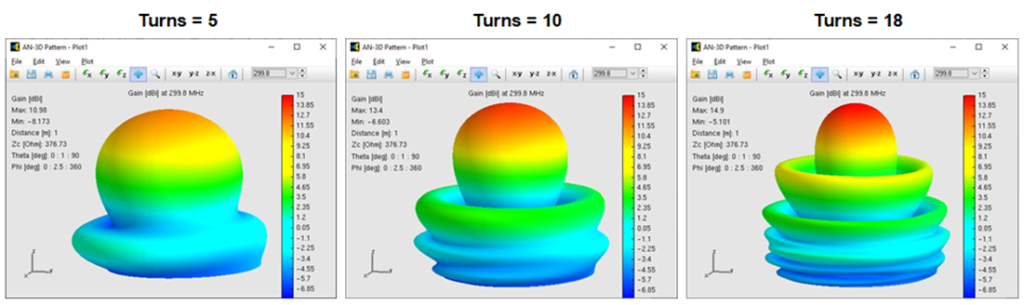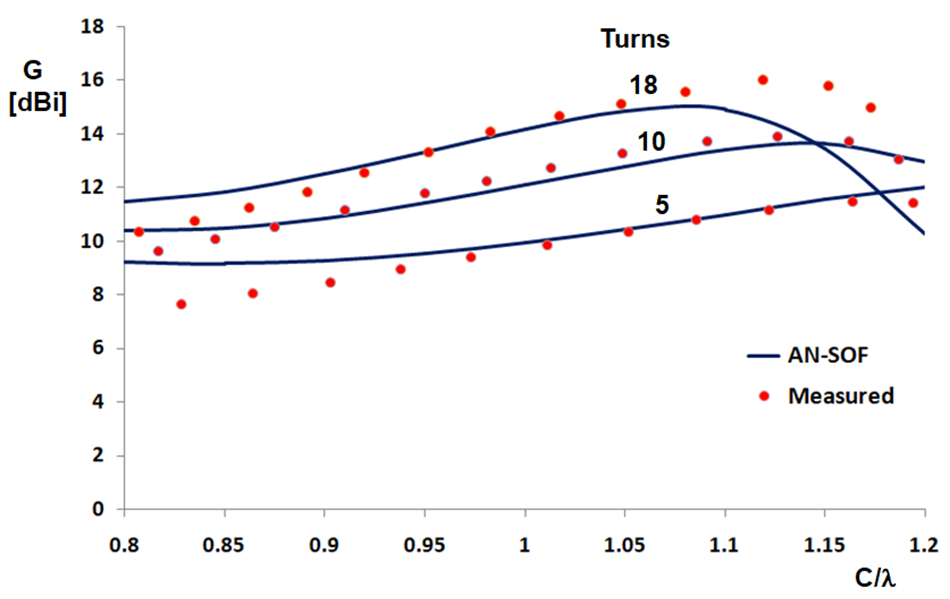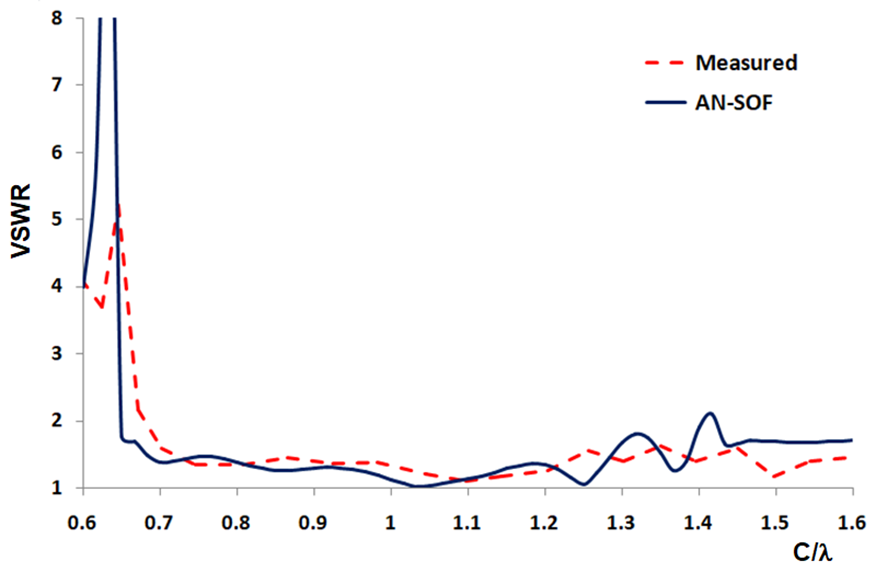How can we help?

# Gain and VSWR of Helix in Axial Mode

A helical antenna operating in axial mode can be a highly directional radiator. Its radiation pattern consists of a main lobe and several secondary lobes, as Fig. 1 shows.Fig. 1: Calculated gain patterns of axial mode helical antennas for different number of turns for C/λ = 1.1 and a pitch angle of α = 12.8°.

The gain increases as the number of turns increases for a certain range on the helix circumference, C/λ. Prof. Krauss has investigated this behavior and reported the measured data shown in Fig. 2, where the calculated results are also plotted. Refer to “Antennas” by John D. Krauss, 2nd Edition, McGraw-Hill, 1988.

Since the details about the experimental setup regarding the feeding system and wire radii involved has not been reported, we have varied the wire radius and the length of the short wire that connects the helix to the ground plane in order to obtain the closest fit for all curves at the same time. We can see that the agreement is quite good along most of the range of C/λ and that the gain maxima occurs around C/λ = 1.1 and 1.2 as Prof. Krauss has observed in his experiments.Fig. 2: Measured and calculated gain curves of axial mode helical antennas as a function of the normalized helix circumference, C/λ, for different number of turns for a pitch angle of α = 12.8°.

Instead of reporting the measured input impedance in detail, the VSWR has been published in Fig. 7-23 of Prof. Krauss book. These data are reproduced in Fig. 3 and compared with the calculated results, where the VSWR is shown as a function of the normalized helix circumference for a 6-turn, 14° helical antenna. We can see that the agreement between the measured and computed results is quite good and that the simulation can reproduce the dip at C/λ = 0.65 as well as the peak structure between 1.2 and 1.5.Fig. 3: Measured and calculated VSWR of 6-turn, 14° axial mode helical antennas as a function of the normalized helix circumference, C/λ.